# How to Do Long Division

Long division is one of the arithmetic basics. It is used when you need to divide multi-digit numbers. This algorithm helps to split the calculation into a few simple steps.

5-Minute Crafts would like to explain to you how to do long division with remainders and without them.

### Division components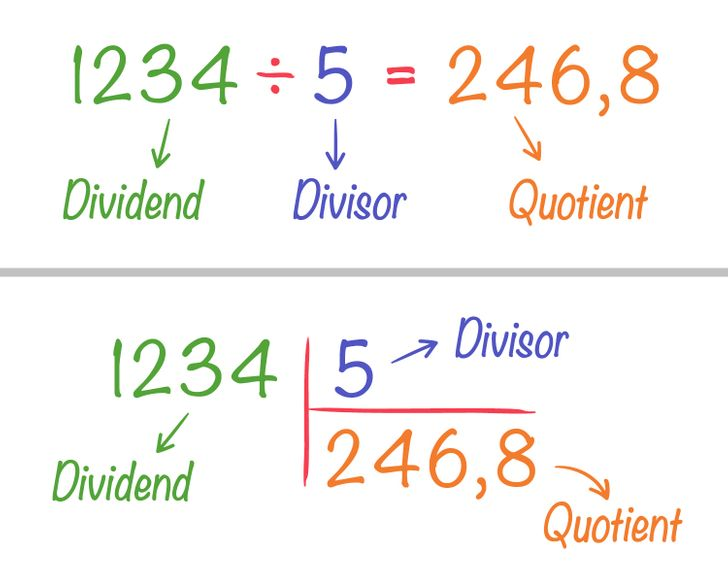• The dividend is a number that we divide.

• The divisor is a number by which another number is to be divided.

• The quotient is the result of the division.

### How to do long division with remainders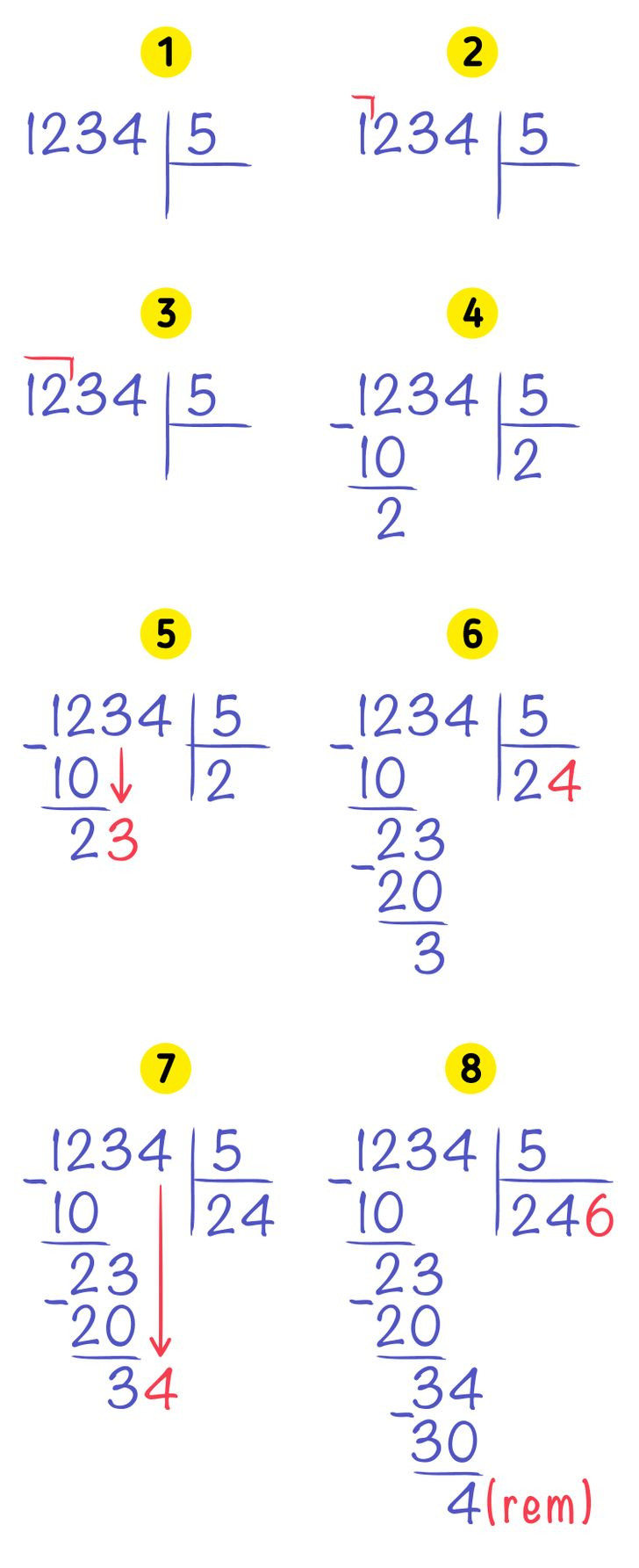1. Write down the dividend on the left and the divisor on the right. Draw a vertical line between them and a horizontal line under the divisor.

2. Start dividing 1,234 by 5. First, compare the numbers of the dividend and the divisor. The first digit in the dividend is 1. 1 is smaller than 5, so you need to take one more digit from the dividend.

3. Compare 12 and 5. 12 is bigger than 5, so we can start dividing.

4. You can’t divide 12 by 5, so you have to calculate how many fives there are in 12. Turn to the multiplication table. 5×2=10. This means that there are 2 fives inside 12. Write 2 under the divisor, and write 10 under 12, then draw a line under 10. Find the difference between these 2 numbers. 12—10=2. Write 2 under the line.

5. Write the next digit of the dividend next to 2. This is 3.

6. Repeat the approach described in step 4, and define how many divisors there are in the dividend, or how many fives there are in 23. 5×4=20. This means that there are 4 fives in the dividend. Write 4 under the divisor and 20 under 23, then draw a line. 23—20=3, so write 3 under the line.

7. Bring down the next digit of the dividend. It’s 4.

8. Repeat the previous steps. 5×6=30, 34—30=4. Write 6 under the divisor and 30 under 34, then draw a line. Write 4 under the line. Since there no more digits in the dividend, you shouldn’t divide further. The answer is 1,234:5=246 (rem. 4).

### Long division without remainders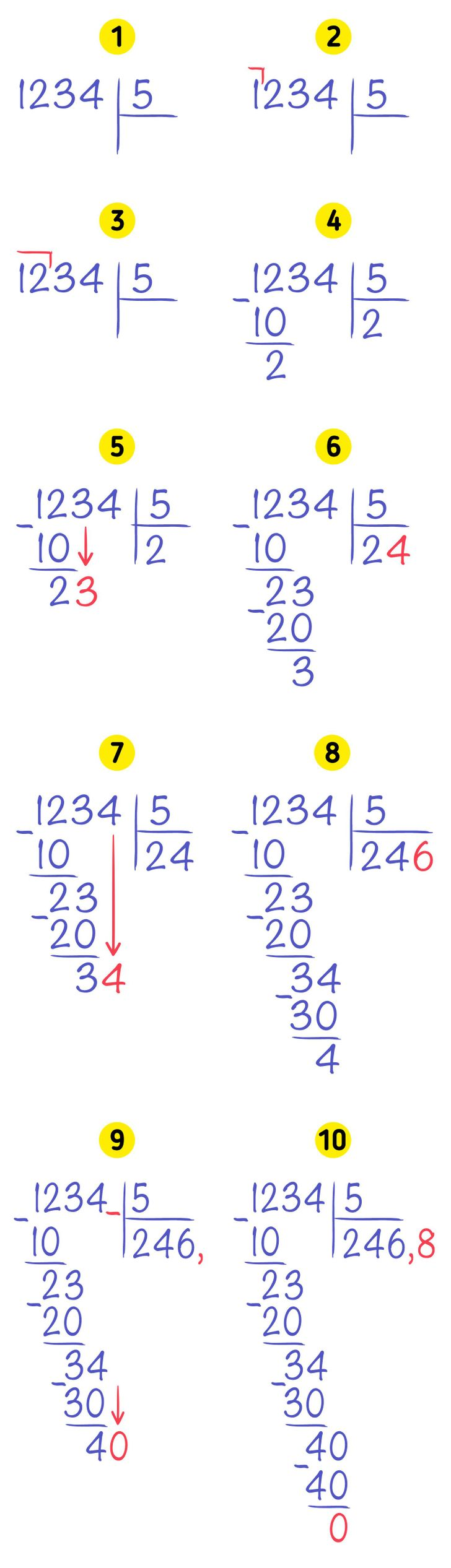Let’s do long division without remainders and use the same example, 1,234:5=? Steps 1 to 7 should be repeated. Let’s start from step 8.

8. Repeat the previous steps. 5×6=30, 34—30=4. Write 6 under the divisor. Write 30 under 34 and draw a line. Write 4 under the line. There no more digits in the dividend, but since we are doing division without remainders, this not the final answer yet.

9. Since there are no more digits in the dividend, put a dot in the quotient. Write 0 next to 4.

10. Now divide 40 by 5. As usual, you need to calculate how many fives there are in 40. 5×8=40, 40—40=0. Write 8 after the dot in the quotient. This is the final answer: 1,234:5=246.8.

### The way to write long division in the USA and the UK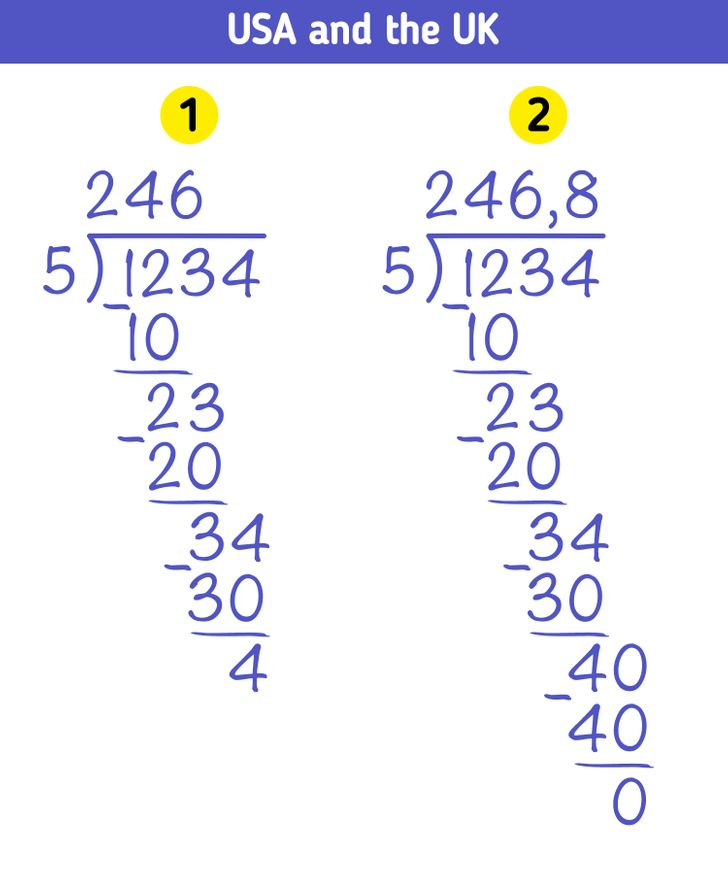In different countries, long division is written down in different ways. In USA and the UK, for example, you have to write the dividend to the right of the divisor, while the quotient is written under the dividend.

### The way to write down long division in Germany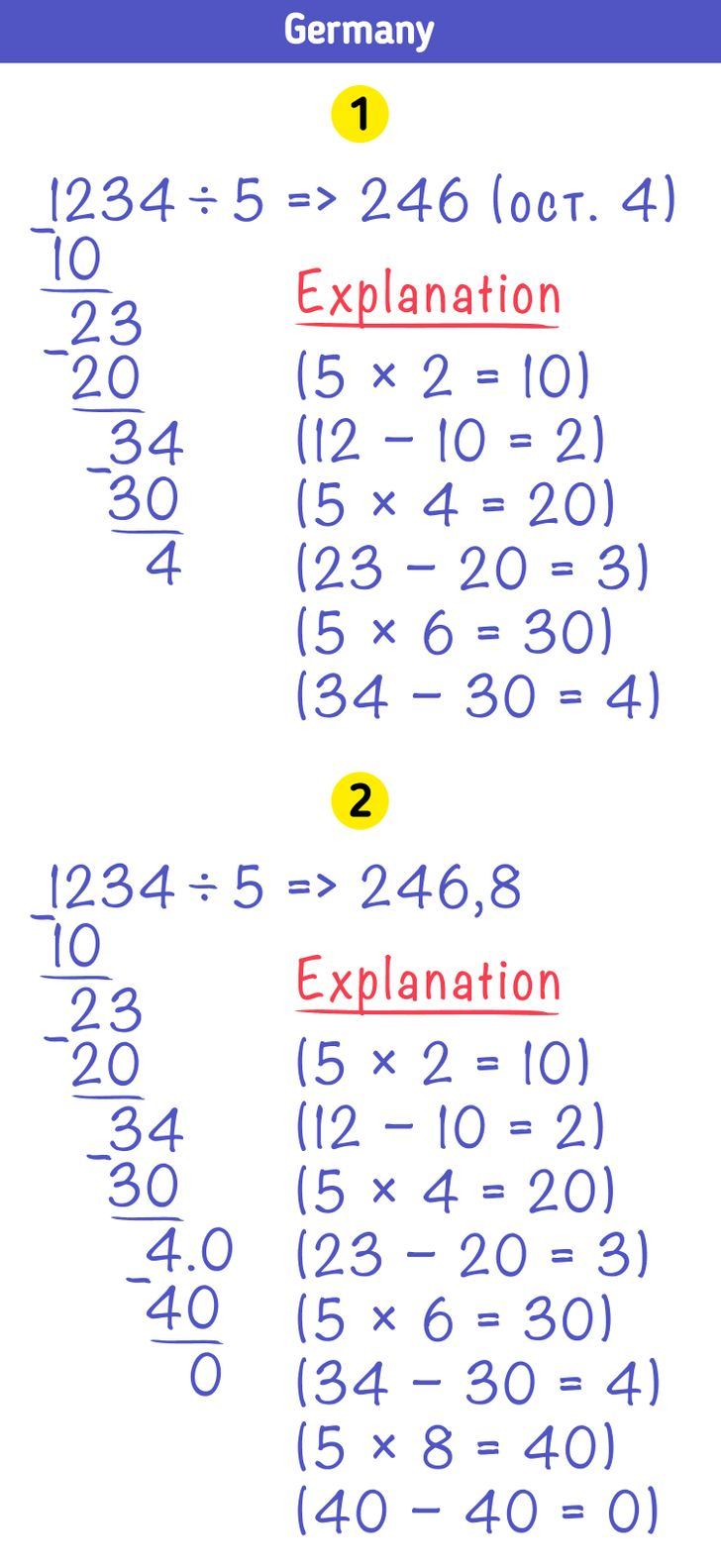In Germany, they write down long division as a normal equation. The calculation is done in a similar way, but it requires an explanation.

### The way to write down long division in the Netherlands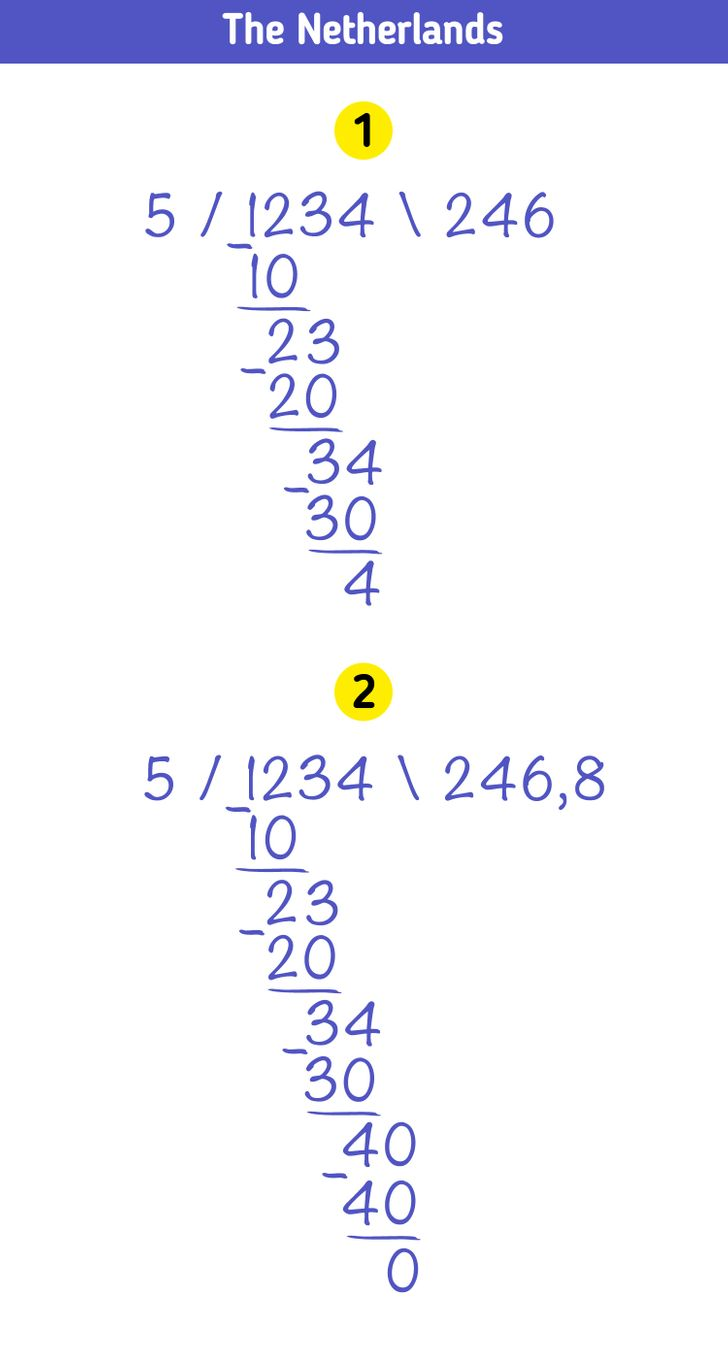In the Netherlands, the process of calculation is no different from the previous options, but it’s written down in a different way. The divisor is put to the left of the dividend, while the quotient is put to the right.

5-Minute Crafts/Life/How to Do Long Division